# Exam 2 Review Review The final exam will

• Slides: 10Exam 2 ReviewReview • The final exam will be comprehensive. • It will cover material in the text book from Chapters 1 – 6. • It will also cover material not in the text book that was provided to you in the lecture notes. • Please see the Exam 1 review for what to expect from chapters 1 -- 6. 2 – All of that information still appliesReview – Discrete Models • Exam 1 – Covered some mathematical and graphical aspects of discrete equations (problem 1) • Exam 2 – Will likely cover modeling aspects of discrete equations • Model development, analysis, and interpretation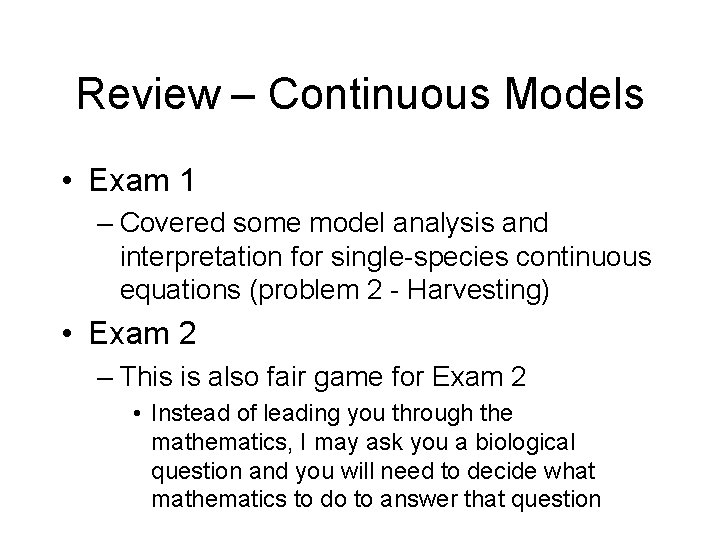Review – Continuous Models • Exam 1 – Covered some model analysis and interpretation for single-species continuous equations (problem 2 - Harvesting) • Exam 2 – This is also fair game for Exam 2 • Instead of leading you through the mathematics, I may ask you a biological question and you will need to decide what mathematics to do to answer that question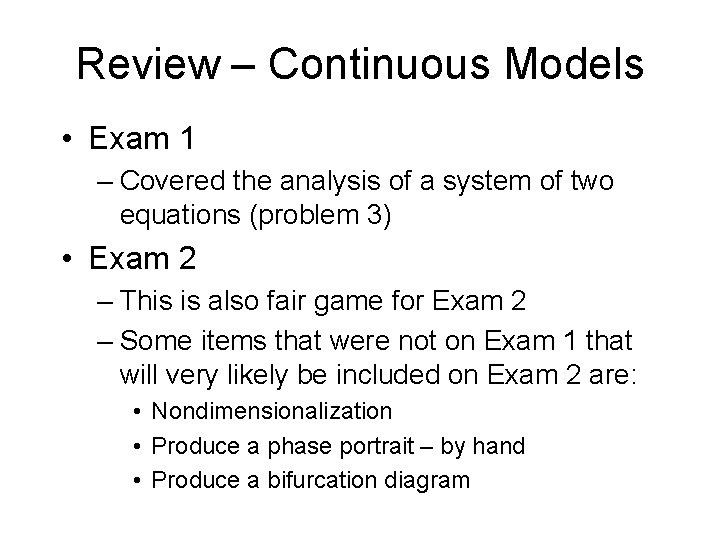Review – Continuous Models • Exam 1 – Covered the analysis of a system of two equations (problem 3) • Exam 2 – This is also fair game for Exam 2 – Some items that were not on Exam 1 that will very likely be included on Exam 2 are: • Nondimensionalization • Produce a phase portrait – by hand • Produce a bifurcation diagram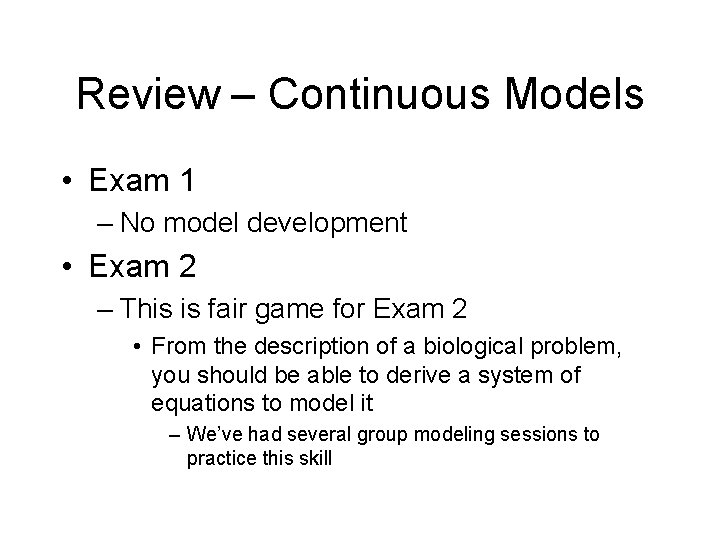Review – Continuous Models • Exam 1 – No model development • Exam 2 – This is fair game for Exam 2 • From the description of a biological problem, you should be able to derive a system of equations to model it – We’ve had several group modeling sessions to practice this skill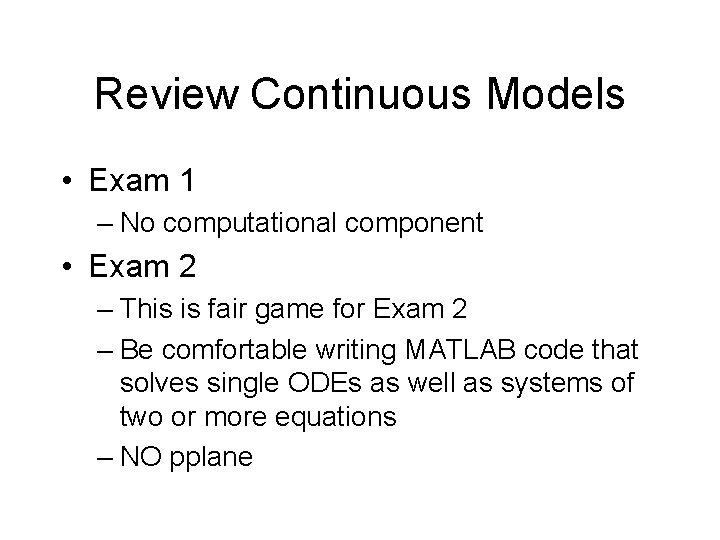Review Continuous Models • Exam 1 – No computational component • Exam 2 – This is fair game for Exam 2 – Be comfortable writing MATLAB code that solves single ODEs as well as systems of two or more equations – NO pplane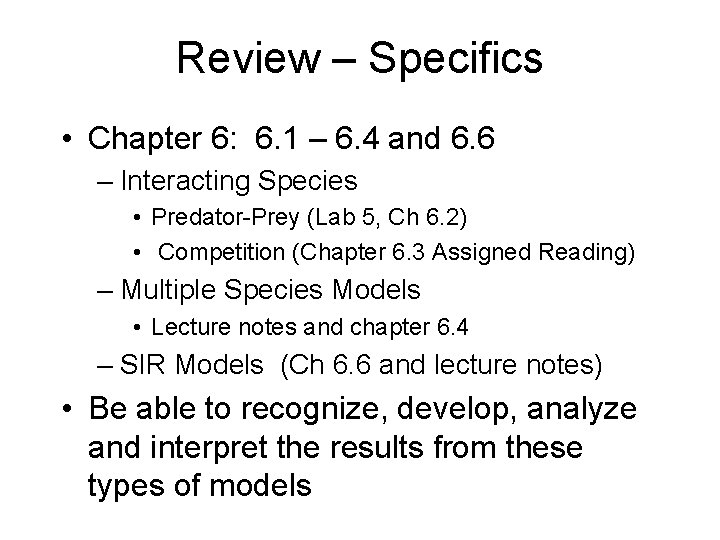Review – Specifics • Chapter 6: 6. 1 – 6. 4 and 6. 6 – Interacting Species • Predator-Prey (Lab 5, Ch 6. 2) • Competition (Chapter 6. 3 Assigned Reading) – Multiple Species Models • Lecture notes and chapter 6. 4 – SIR Models (Ch 6. 6 and lecture notes) • Be able to recognize, develop, analyze and interpret the results from these types of models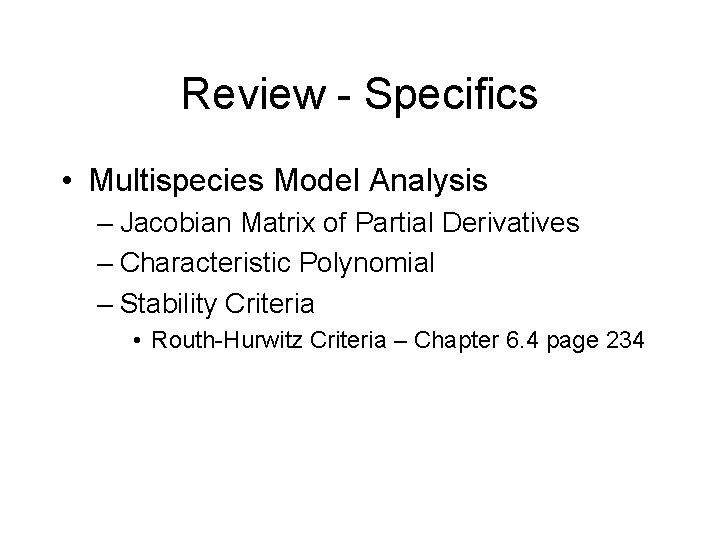Review - Specifics • Multispecies Model Analysis – Jacobian Matrix of Partial Derivatives – Characteristic Polynomial – Stability Criteria • Routh-Hurwitz Criteria – Chapter 6. 4 page 234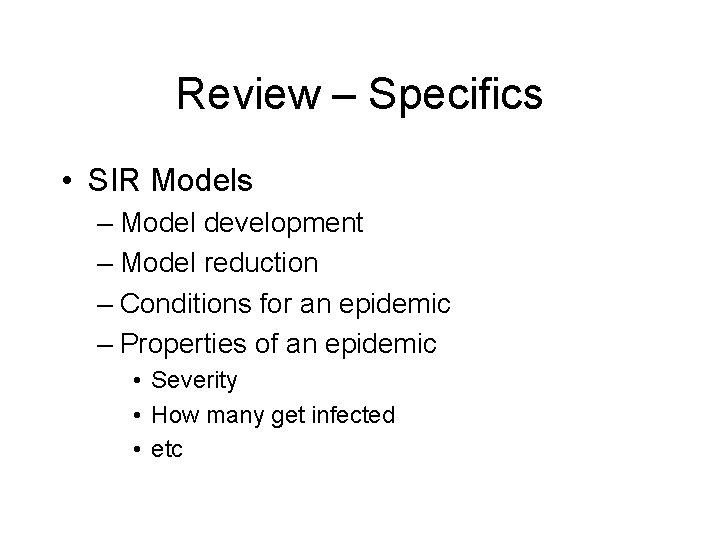Review – Specifics • SIR Models – Model development – Model reduction – Conditions for an epidemic – Properties of an epidemic • Severity • How many get infected • etc